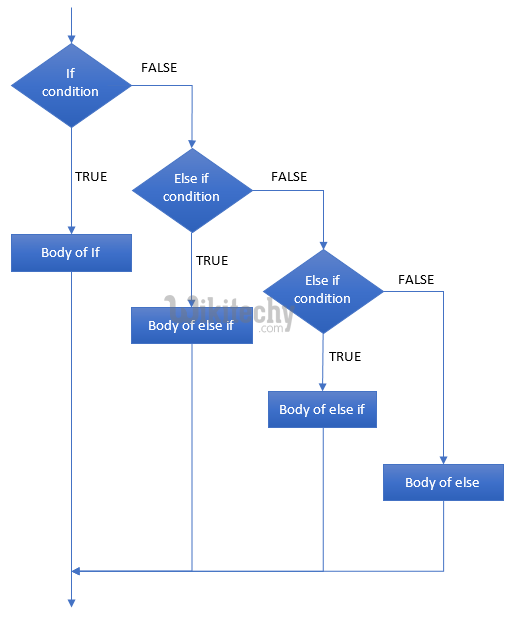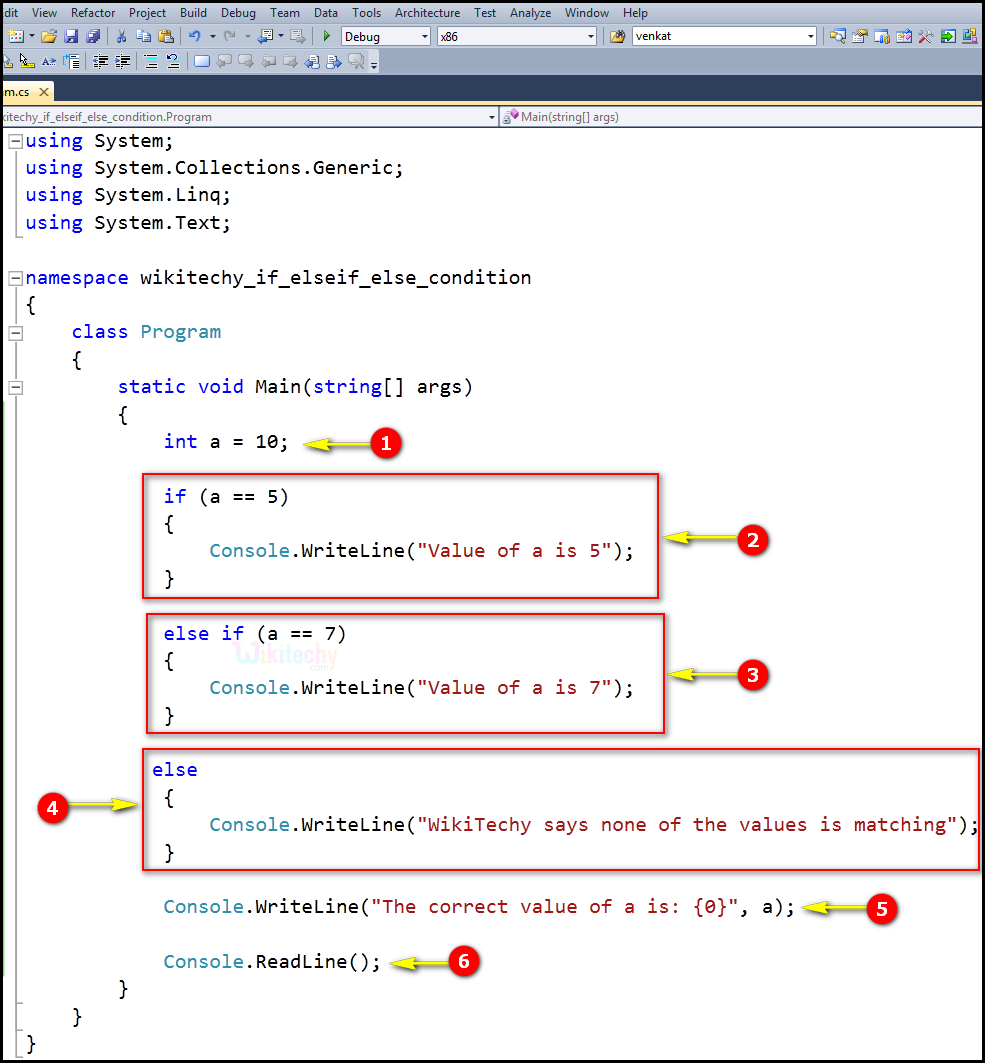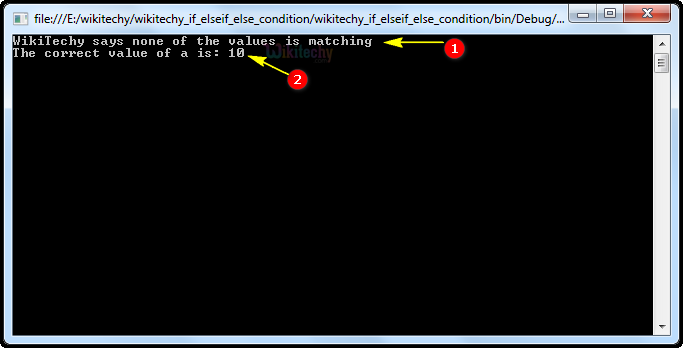# C# else if | if else c# | c# if - c# - c# tutorial - c# netC# Else If

## What is else if statement in C# ?

• In C#, an if statement can be followed by an optional else if...else statement.
• And these statement is very useful to test various conditions using single in if...else if statement.## Code Explanation:1. a = 10 is an integer variable value as 10.
2. if (a == 5) specifies to If the condition is true (10 == 5) then the control goes to the body of if block, that is the program will execute the "Value of a is 5".
3. else if (a == 7) specifies to if else if condition is true (10 == 7) then the control goes to the body of else if block, that is the program will execute the "Value of a is 7". (where the elseif condition is false it go to else condition).
4. If the condition is false then the control goes to next level, that is if we provide else block the program will execute the else statement "WikiTechy says none of the values is matching".
5. In this example Console.WriteLine, the Main method specifies its behavior with the statement "The correct value of a is". And WriteLine is a method of the Console class defined in the System namespace. This statement reasons the message "The correct value of a is" to be displayed on the screen.
6. Here Console.ReadLine(); specifies to reads input from the console. When the user presses enter, it returns a string.

## Sample C# examples - Output :1. Here in this output statement executes the else condition by printing the statement "WikiTechy says none of the values is matching".
2. Here in this output we display "The correct value of a is 10". Which specifies to output console application.